### Problems in BackPropagation and Gradient Descent

1. the gradient of reward signals given to the agent is realised many timesteps in the future. Questions above can be seem as Credit Assignment

2. there is the issue of being stuck in a local optimum.### Pseudo code of Basic Evolution Strategy

1. Easier to scale in a distributed setting(easy to parallelize).
2. It does not suffer in settings with sparse rewards.
3. It has fewer hyperparameters.
4. It is effective at finding solutions for RL tasks.### Improvement of Covariance Matrix Adaptive Evolution Strategy

We want to explore more and increase the standard deviation of our search space.
And there are times when we are confident we are close to good optima and just want to fine-tune the solution.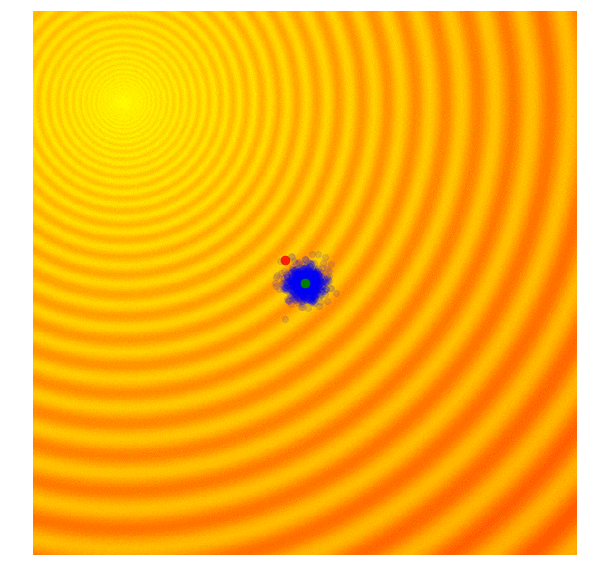### Details of algorithm

1. Calculate the fitness score of each candidate solution in generation $(g)$.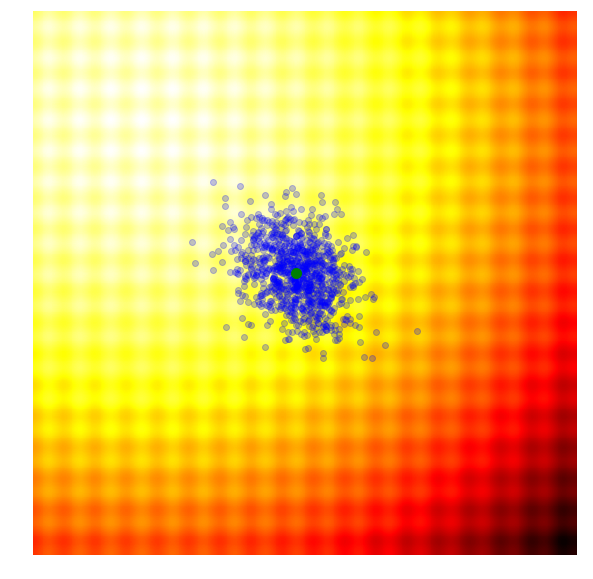2. Isolates the best 25% of the population in generation $(g)$, in purple.1. Using only the best solutions, along with the mean $\mu^{(g)}$ of the current generation (the green dot), calculate the covariance matrix $C^{(g+1)}$ of the next generation.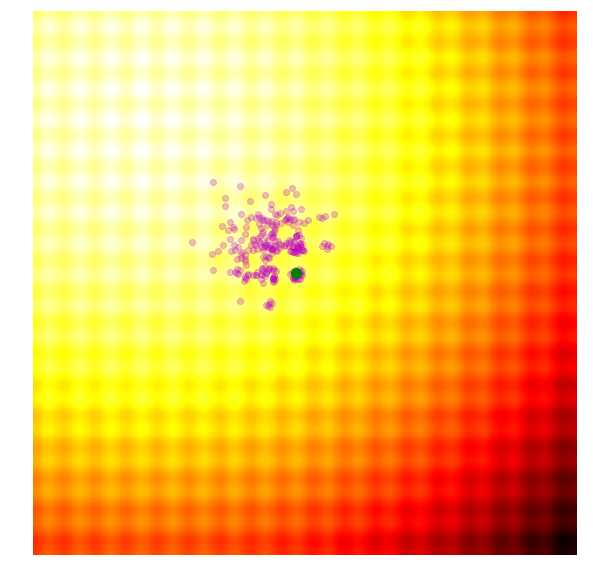1. Sample a new set of candidate solutions using the updated mean $\mu^{(g+1)}$ and covariance matrix $C^{(g+1)}​$.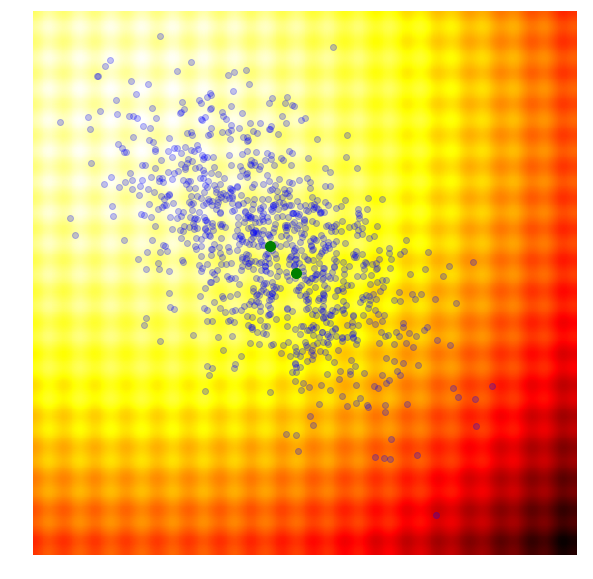### OpenAI Evolution Strategy

In particular, $\sigma$ is fixed to a constant number, and only the $\mu$ parameter is updated at each generation.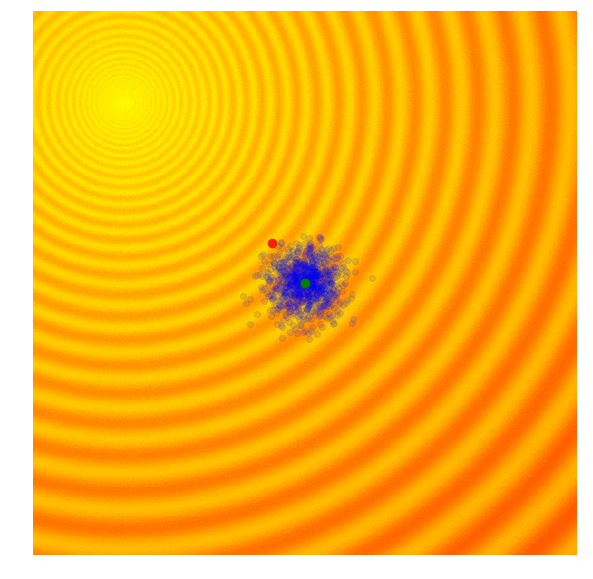Although its performance is not the best, it is possible to scale to over a thousand parallel workers.### Comparision among Evolution Strategies0%